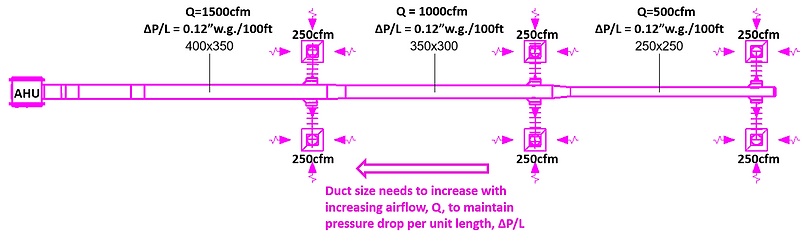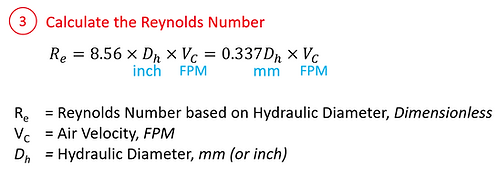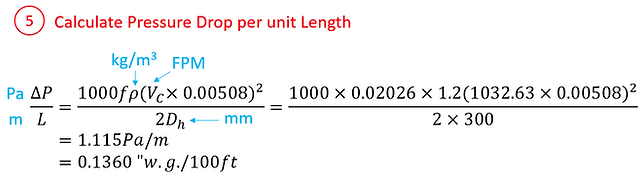Duct Sizer and Pressure Drop Calculator – Computing Pressure Drop due to Friction for a straight length of Duct

Duct sizing is generally done in 2 ways: through the velocity reduction method, and the equal friction method.

The equal friction method determines the duct size by assuming a constant pressure loss per unit length allowed, say, 0.12” w.g. per 100 feet of duct, or 1 Pa per meter run.

As elaborated below, a set of formulae relates airflow (CFM or ft3/min) to duct loss per unit length (“w.g./100 ft). Based on increasing airflow along the duct after each branch (or take-offs) nearer to the unit, the designed duct size shall increase so that the pressure loss per unit length remains consistent throughout.To relate airflow (CFM) to duct loss per unit length (Pa/m), the following formula derived from Altschul-Tsal’s equation may be used for the approximation with reference to Colebrook’s equation (of course, other equations may also be applicable). It may look complicated when represented in a single equation below, but it will turn out to be simple as we go through the parameters step-by-step afterward.The above equations that relates airflow (CFM) to duct loss per unit length (Pa/m) can be represented with the excel equation below.

=1000*IF(0.11*(D2/(4*D4*D5/(D4+D5)/2)+68/(0.337*(4*D4*D5/(D4+D5)/2)*(D1/(D4/1000*D5/1000*10.76))))^0.25<0.018,0.11*(D2/(4*D4*D5/(D4+D5)/2)+68/(0.337*(4*D4*D5/(D4+D5)/2)*(D1/(D4/1000*D5/1000*10.76))))^0.25*0.85+0.0028,0.11*(D2/(4*D4*D5/(D4+D5)/2)+68/(0.337*(4*D4*D5/(D4+D5)/2)*(D1/(D4/1000*D5/1000*10.76))))^0.25)*D3*((D1/(D4/1000*D5/1000*10.76))*0.00508)^2/(2*(4*D4*D5/(D4+D5)/2))

Where:

D1          = Airflow, Q (mm)

D2          = Absolute Roughness, ε (mm) (ε=0.15mm for Galvanised Iron)

D3          = Density of Air, ρ (kg/m3) (ρ=1.2041 kg/m3 for air at 20 °C and 101.325 kPa)

D4          = Duct Width, W (mm)

D5          = Duct Height, H (mm)

You may “Find” and “Replace All” values for D1 to D6 to your respective input cells in Excel, or download the Excel file below to work on.

You may refer to Engineering Toolbox for absolute roughness based on different materials, or refer to the tabulation below:

Relating Pressure Drop ("w.g./100ft) to Airflow (cfm) (Excel)To summarise, the above equation can be established with the simple 5 steps elaborated below. To minimise confusion, the units that shall be used for each of the steps were indicated in cyan beside each variable.

1. Calculate Air Velocity, VC

2. Calculate Hydraulic Diameter, Dh

3. Calculate Reynolds Number, Re

4. Calculate the Friction Factor, f

5. Calculate the Pressure Drop per unit Length, ΔP/LBelow summarises some of the key conversions which may be useful in the above derivations.

Worked Example:

Determine the Frictional Pressure Drop for G.I. duct of size 300x300, 10 meters long, carrying 1,000 cfm at approx. 20 deg.

Q            = 1000 cfm

ε             = 0.15 mm for G.I.

W           = 300 mm

H            = 300 mm

ρ             = 1.2 kg/m3 at 20 °CReverse Engineering: Computing Duct Size from Allowable Pressure Drop

The other way around, Computing Duct Size from Allowable Pressure Drop can be much more complicated. To name a few methods, it can either be done with Excel Solver, or by tabulating large samples of pressure losses with different duct sizes, and then use interpolation to match the right size.

The following downloadable excel file shows the latter method. while it is free for download, we also produced a premium version of duct sizer with the following enhancement. Note that this duct sizer shall be macro-enabled as VBA macros are involved.

Enhancements of Premium Version of Duct Sizer

2. Ability to toggle between "Metric" and "Imperial" unit with a single click. Units conversion applies for all cells including those from user inputs

3. No protected cells/Sheets

4. Ability to compute duct size, or override duct size manually for a computed loss information

5. Ability to toggle between commonly available, rounded-up values of duct size, or using the exact values.

6. Ability to compute based on both sizing methods: Pressure Drop or Air Velocity.

7. Ability to compute loss for both rectangular or round duct.

8. Ability to unhide all "workings" cells for trouble shooting, and re-hide again thereafter.

Duct Sizer (No Macro required)The online duct static pressure drop calculator sums up the steps in the example shown above for the computation of pressure drop per unit length, ΔP/L ("w.g./100ft)